# Solving Derivatives Flashcards

Solving Derivatives Flashcards
1/26 (missed) 0 0
Create Your Account To Continue Studying

As a member, you'll also get unlimited access to over 75,000 lessons in math, English, science, history, and more. Plus, get practice tests, quizzes, and personalized coaching to help you succeed.

Try it risk-free for 30 days. Cancel anytime.
###### Already registered? Login here for access
Find the second derivative of y = cos(x)
The second derivative is -cos(x)
Got it
Find the derivative of g(x) = cos(2x)
The derivative g'(x) = -2 sin(2x)
Got it
Find the derivative of f(x) = 3 ln(x)
The derivative f '(x) = 3 / x
Got it
Find the derivative of h(x) = 2x3 + 4x2 - x - 3
The derivative is h'(x) = 6x2 + 8x - 1
Got it
Find d/dx cos(x)
d/dx cos(x) = -sin(x)
Got it
State the relationship between a position function in terms of t and the acceleration function in terms of t.
The acceleration function is the second derivative of the position function.
Got it
Find f '(x) for f(x) = cos-1 (x)
The derivative is: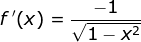Got it
Determine the derivative of y = y2 - 2xy
The derivative is: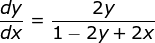Got it
Find d/dx sin(x)
d/dx sin(x) = cos(x)
Got it
Find the fourth derivative of g(x) = x6
The fourth derivative is 360x2
Got it
Product Rule for Differentiation
The derivative of f(x)*g(x) is f(x)*g'(x) + g(x)*f '(x)
Got it
Determine the derivative of g(x) = 3(x3 - 4x2 + 2)3
The derivative is g'(x) = 9(3x2 - 8x)(x3 - 4x2 + 2)2
Got it
Find the derivative of f(x) = 2x5 + ex - 3x - 6
The derivative is f'(x) = 10x4 + ex - 3
Got it

### Ready to move on?

or choose a specific lesson: See all lessons in this chapter
26 cards in set

## Flashcard Content Overview

These flashcards can help you practice many of the concepts of differentiation. Derivatives of polynomial, exponential, and trigonometric functions are covered. The Chain Rule, implicit differentiation, and higher order derivatives are also reviewed.

Front
Back
Find the derivative of f(x) = 2x5 + ex - 3x - 6
The derivative is f'(x) = 10x4 + ex - 3
Determine the derivative of g(x) = 3(x3 - 4x2 + 2)3
The derivative is g'(x) = 9(3x2 - 8x)(x3 - 4x2 + 2)2
Product Rule for Differentiation
The derivative of f(x)*g(x) is f(x)*g'(x) + g(x)*f '(x)
Find the fourth derivative of g(x) = x6
The fourth derivative is 360x2
Find d/dx sin(x)
d/dx sin(x) = cos(x)
Determine the derivative of y = y2 - 2xy
The derivative is:Find f '(x) for f(x) = cos-1 (x)
The derivative is:State the relationship between a position function in terms of t and the acceleration function in terms of t.
The acceleration function is the second derivative of the position function.
Find d/dx cos(x)
d/dx cos(x) = -sin(x)
Find the derivative of h(x) = 2x3 + 4x2 - x - 3
The derivative is h'(x) = 6x2 + 8x - 1
Find the derivative of f(x) = 3 ln(x)
The derivative f '(x) = 3 / x
Find the derivative of g(x) = cos(2x)
The derivative g'(x) = -2 sin(2x)
Find the second derivative of y = cos(x)
The second derivative is -cos(x)
Find the third derivative of y = 2x4 - 3x2
The third derivative is 48x
Find the fourth derivative of y = 2x2
The fourth derivative is 0
Find the seventh derivative of g(x) = 3ex
The seventh derivative is 3ex
Find the derivative of y = 3
The derivative y ' = 0
Differentiate f(x) = 4x3 - 5x2 + x
f '(x) = 12x2 - 10x + 1
Find the derivative of f(x) = (2x + 5)3
f '(x) = 6(2x + 5)2
You throw a pebble into a still pond. If the radius of the circular waves increases by 10 cm per second, how fast is the area of the circle increasing?
The area is increasing by 20pi cm2/sec.
Differentiate: y = cos2 (5 - 3x)
The derivative is: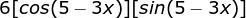Find the second derivative: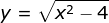The second derivative is: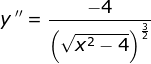Problem: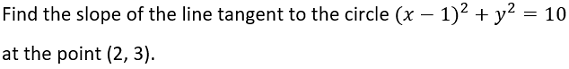The slope is -1/3
Differentiate f(x) = cos2 (5x)
f '(x) = -10 cos(5x) sin(5x)
Differentiate: 3y = 4xy - 7x
The derivative is: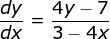Use implicit differentiation to find dy/dx of 3x - 4y = 2 cos(y) + 7x
The implicit derivative is: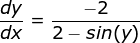To unlock this flashcard set you must be a Study.com Member.